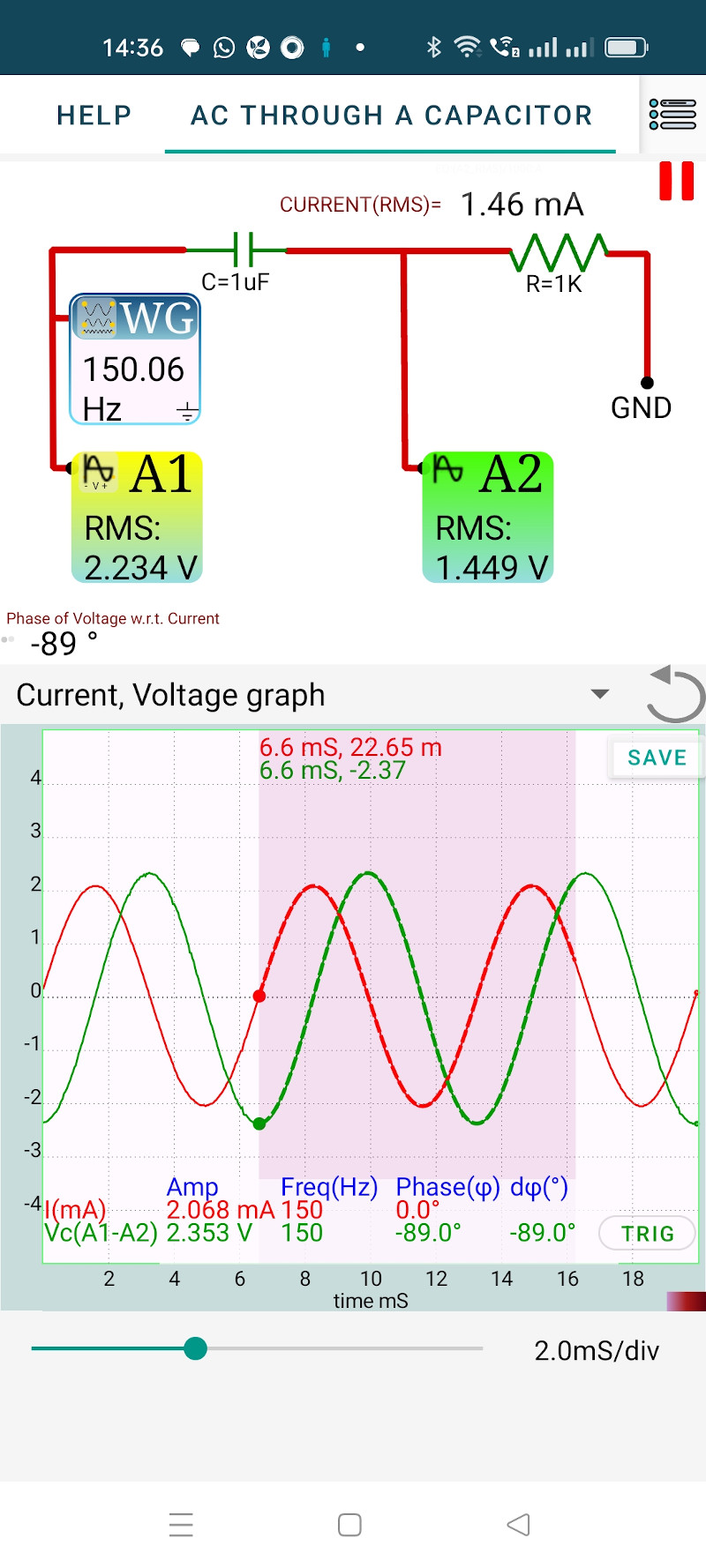# ExpEYES

## Capacitor in AC Circuits

The objective of this experiment is to explore the phase relatioships of voltage and current across a capacitor in an AC circuit. We also calculate the capacitive reactance from the current and voltage, using Ohm’s law. The 1K resistor and A2 constitutes our ammeter.

• Make the connections as shown in the figure.
• 1 uF capacitor in series with a 1 kOhm resistor.
• Connect the capacitor end to WG
• Connect the resistor end to ground
• Connect A1 to WG
• Connect A2 to the junction between R and C
• Adjust the timebase to view 3 or 4 cycles
• Select a region, 2 or 3 cycles, by sliding horizontally.The amplitude, frequency and phase are displayed when a region is selected. It can be seen that the current is at the peak when the voltage across the capacitor is at zero, means the voltage is lagging by 90 degrees.

• The peak voltage across the capacitor = 2.353 volts
• Current though the circuit = 2.068 mA
• Frequency = 150.06 Hz

Capacitive reactance, $X_C=\frac{V_C}{I_C}=\frac{1}{2\pi fC}$, implies $C=\frac{I_C}{V_C 2\pi f }$ = 932.5 uF

Measurement of the capacitor by using IN1 gives 937 uF. This confirms that the results agree with the theory within the experimental error.